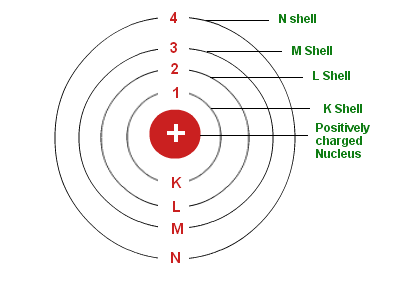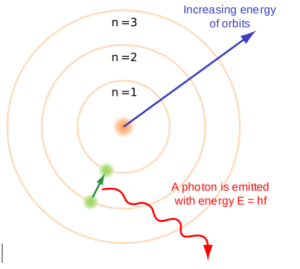# Bohr's Model Of An Atom

## Introduction to the Bohr Model

Bohr model of the atom was proposed by Neil Bohr in 1915. It came into existence with the modification of Rutherford’s model of an atom. Rutherford’s model introduced the nuclear model of an atom, in which he explained that a nucleus (positively charged) is surrounded by negatively charged electrons.

Bohr modified this atomic structure model by explaining that electrons move in fixed orbital’s (shells) and not anywhere in between and he also explained that each orbit (shell) has a fixed energy level. Rutherford basically explained the nucleus of an atom and Bohr modified that model into electrons and their energy levels.Bohr’s Model of an Atom

Bohr’s model consists of a small nucleus (positively charged) surrounded by negative electrons moving around the nucleus in orbits. Bohr found that an electron located away from the nucleus has more energy, and electrons close to the nucleus have less energy.

## Postulates of Bohr’s Model of an Atom

• In an atom, electrons (negatively charged) revolve around the positively charged nucleus in a definite circular path called as orbits or shells.
• Each orbit or shell has a fixed energy and these circular orbits are known as orbital shells.
• The energy levels are represented by an integer (n=1, 2, 3…) known as the quantum number. This range of quantum number starts from nucleus side with n=1 having the lowest energy level. The orbits n=1, 2, 3, 4… are assigned as K, L, M, N…. shells and when an electron attains the lowest energy level it is said to be in the ground state.
• The electrons in an atom move from a lower energy level to a higher energy level by gaining the required energy and an electron moves from a higher energy level to lower energy level by losing energy.## Limitations of Bohr’s Model of an Atom

• Bohr’s model of an atom failed to explain the Zeeman Effect (effect of magnetic field on the spectra of atoms).
• It also failed to explain the Stark effect (effect of electric field on the spectra of atoms).
• It violates the Heisenberg Uncertainty Principle.
• It could not explain the spectra obtained from larger atoms.

## Recommended Videos## FAQs

1. How do electrons move in Bohr’s model?
Ans: The theory notes that electrons in atoms travel around a central nucleus in circular orbits and can only orbit stably at a distinct set of distances from the nucleus in certain fixed circular orbits. Such orbits are related to certain energies and are also referred to as energy shells or energy levels.

2. How did Bohr discover electrons?
Ans: Bohr was the first to discover that electrons move around the nucleus in different orbits and that an element’s properties are determined by the number of electrons in the outer orbit.

3. Did Bohr’s model have neutrons?
Ans: The nucleus in the atom’s Bohr model holds most of the atom’s mass in its protons and neutrons. The negatively charged electrons, which contribute little in terms of mass, but are electrically equivalent to the protons in the nucleus, orbit the positively charged core.

4. How did Sommerfeld modify Bohr’s theory?
Ans: Many modifications have been introduced to the Bohr model, most notably the Sommerfeld model or Bohr – Sommerfeld model, which suggested that electrons move around a nucleus in elliptical orbits rather than circular orbits of the Bohr model. The Bohr – Sommerfeld system was essentially incoherent, contributing to many paradoxes.

5. Who discovered electrons?
Ans: J. J. Thomson in 1897 discovered Electron when he was studying the properties of the cathode ray.